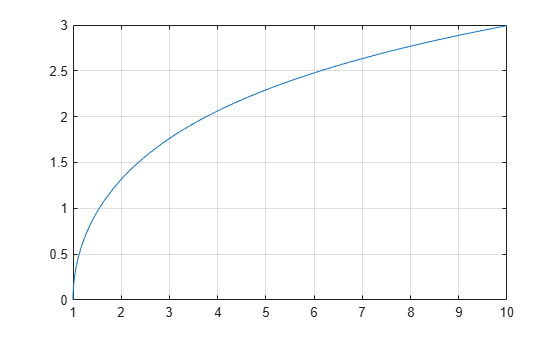acosh

Symbolic inverse hyperbolic cosine function

Description

example

acosh(X) returns the inverse hyperbolic cosine function of X.

Examples

Inverse Hyperbolic Cosine Function for Numeric and Symbolic Arguments

Depending on its arguments, acosh returns floating-point or exact symbolic results.

Compute the inverse hyperbolic cosine function for these numbers. Because these numbers are not symbolic objects, acosh returns floating-point results.

A = acosh([-1, 0, 1/6, 1/2, 1, 2])
A =
0.0000 + 3.1416i   0.0000 + 1.5708i   0.0000 + 1.4033i...
0.0000 + 1.0472i   0.0000 + 0.0000i   1.3170 + 0.0000i

Compute the inverse hyperbolic cosine function for the numbers converted to symbolic objects. For many symbolic (exact) numbers, acosh returns unresolved symbolic calls.

symA = acosh(sym([-1, 0, 1/6, 1/2, 1, 2]))
symA =
[ pi*1i, (pi*1i)/2, acosh(1/6), (pi*1i)/3, 0, acosh(2)]

Use vpa to approximate symbolic results with floating-point numbers:

vpa(symA)
ans =

[ 3.1415926535897932384626433832795i,...
1.5707963267948966192313216916398i,...
1.4033482475752072886780470855961i,...
1.0471975511965977461542144610932i,...
0,...
1.316957896924816708625046347308]

Plot Inverse Hyperbolic Cosine Function

Plot the inverse hyperbolic cosine function on the interval from 1 to 10.

syms x
fplot(acosh(x),[1 10])
grid onHandle Expressions Containing Inverse Hyperbolic Cosine Function

Many functions, such as diff, int, taylor, and rewrite, can handle expressions containing acosh.

Find the first and second derivatives of the inverse hyperbolic cosine function. Simplify the second derivative by using simplify.

syms x
diff(acosh(x), x)
simplify(diff(acosh(x), x, x))
ans =
1/((x - 1)^(1/2)*(x + 1)^(1/2))

ans =
-x/((x - 1)^(3/2)*(x + 1)^(3/2))

Find the indefinite integral of the inverse hyperbolic cosine function. Simplify the result by using simplify.

int(acosh(x), x)
ans =
x*acosh(x) - (x - 1)^(1/2)*(x + 1)^(1/2)

Find the Taylor series expansion of acosh(x) for x > 1:

assume(x > 1)
taylor(acosh(x), x)
ans =
(x^5*3i)/40 + (x^3*1i)/6 + x*1i - (pi*1i)/2

For further computations, clear the assumption on x by recreating it using syms:

syms x

Rewrite the inverse hyperbolic cosine function in terms of the natural logarithm:

rewrite(acosh(x), 'log')
ans =
log(x + (x - 1)^(1/2)*(x + 1)^(1/2))

Input Arguments

collapse all

Input, specified as a symbolic number, variable, expression, or function, or as a vector or matrix of symbolic numbers, variables, expressions, or functions.